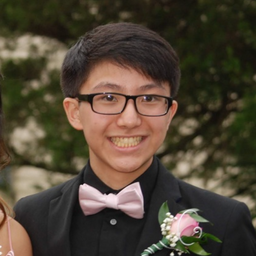## = e-2

### Discussion

You must be signed in to discuss.
##### Top Calculus 3 Educators##### Lily A.

Johns Hopkins University##### Kristen K.

University of Michigan - Ann Arbor##### Samuel H.

University of Nottingham

### Video Transcript

all right. So this problem is, I think, one of the more more enjoyable ones. It's a little fun to figure out what to do and how to solve it. And the problem tries to trick us a little bit by giving us this double integral of region are of the function to exit third times each that x squared times supply dx dy y, and it gives us it in the DX dy y form, which is the trick here because it's trying to get you to do in the xy y integral. But that is horrible. You should not do it that way because you might be able to do it that way. But it's probably gonna be a lot harder. Do that way in our region are sorry our region are is defined to be the set of all points X and y that air basically elements or art cornet pairs such that X and y are both between one. Okay, so let's draw out the region Our first it's basically just gonna look like this. This region right here, this square fusion. This is why this sex Um now the trick here is we don't want to do a dxc white. And we actually want to a d y dx in a rule. So each x square. Why d y d x on? We just want to think about out each of these variables range. Why ranges from 01 on that x ray. Just one. Okay. And in order to evaluate this integral, we want to evaluate the inside first. And trust me, this took me a little bit to figure out. So don't worry if you had a little trouble, I figure out how to do this, but you want to a u substitution here, So you let u equal, uh, X squared times. Why? And then since we're in green respect to Y first we have to you is equal to X squared. D y okay. And before we plug in the used stuff first, what I'm gonna do is I'm gonna just take out that eight to acts first. So we're left with X squared here. Usually X. Where times. Why do you Why? And we're allowed to do this because we know that we're interest, but toe accent next. So we know that this two X is going to result in a numeric value after I integrated respect to that. So that's OK for now. So now our focus is on away. I wrote this wrong or today? Uh, no, I didn't. OK, OK, so our focus is on this inner integral. So we have two x times the role of I wanna plug everything in. So we have each of the U and then x squared ey is just do you in this case and then our new limits, We're gonna be what we get from playing in, um, zero for y and one for wife. So when you play in zero, we get zero. And when we plug in, why? For one for why we get X squared, Okay. And then we want to body weight. This we have the anti derivative of each of the you respect to you is just to the U then from zero to x squared. This should be two x times each x squared minus eat zero, which is just two x times these x squared minus one. So this is a result of the inside. Now, what we need to do is plug this into the outside integral. So the girl 01 of two x times use the X where minus one d X. And in order to evaluate this, um, again, we're going to use U substitution. So we like you equal next squared and the D. U is going to be two x dx and then plugging this back into our original integral, we get the integral from it's gonna be Do you so just replaces to extend DX with do you and then each the, you know, minus one. And then our new limits are gonna be zero and one because x square are zero squared to zero and one squared is one And then evaluating this, the entire group of you to you, is he to you? And then the entire group one is thank you and then plugging in one we get eats the one the minus one, the minus each the 00 This guy just be e minus one minus one, which is equal to e minus two. And that is our final cancerRutgers, The State University of New Jersey
##### Top Calculus 3 Educators##### Lily A.

Johns Hopkins University##### Kristen K.

University of Michigan - Ann Arbor##### Samuel H.

University of Nottingham